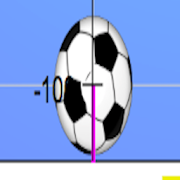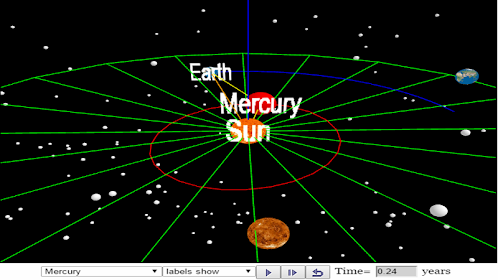# Free Fall SimulatorEveryone
an open source physics at Singapore simulation based on codes written by Loo Kang WEE.

more simulation are available here
http://iwant2study.org/ospsg/index.php/interactive-resources/physics/02-newtonian-mechanics/02-dynamics

Introduction
Topics

Kinematics
Speed, velocity and acceleration
Graphical analysis of motion

Sample Learning Goals
(e) plot and interpret a displacement-time graph and a velocity-time graph
(f) deduce from the shape of a displacement-time graph when a body is:
(i) at rest example of progressive mathematical model is encouraged X = 0
(ii) moving with uniform velocity example of progressive mathematical model is encouraged X = 1*tf or a constant velocity motion of v =1 m/s
(iii) moving with non-uniform velocity example of X = 0.5*1*t^2 for a constant acceleration motion of a =1 m/s^2
(g) deduce from the shape of a velocity-time graph when a body is:
(i) at rest
(ii) moving with uniform velocity
(iii) moving with uniform acceleration
(iv) moving with non-uniform acceleration

Interesting Fact 2
This app is produce real numbers to coincident with the real world data of gravity pull at 9.81 m/s^2 with some random errors simulating real data-logger results. There is a mathematical modelling pedagogy built into the simulation for students to make effort to represent the physics similar to Tracker's particle modeling.

Acknowledgement
My sincere gratitude for the tireless contributions of Francisco Esquembre, Fu-Kwun Hwang, Wolfgang Christian, Félix Jesús Garcia Clemente, Anne Cox, Andrew Duffy, Todd Timberlake and many more in the Open Source Physics community. I have designed much of the above based on their ideas and insights, and I thank the OSP community for which Singapore was honored with 2015-6 UNESCO King Hamad Bin Isa Al-Khalifa Prize for the Use of ICTs in Education.

Network Learn together?
Blog: http://weelookang.blogspot.sg/
Digital Library: http://iwant2study.org/ospsg/
Collapse

Review Policy

## What's New

v5

v4

added new features, test questions, better graphics
Collapse

Updated
March 8, 2017
Size
4.8M
Installs
500+
Current Version
0.0.5
Requires Android
4.1 and up
Content Rating
Everyone
Interactive Elements
Digital Purchases
Permissions
Offered By
Open Source Physics Singapore
Developer